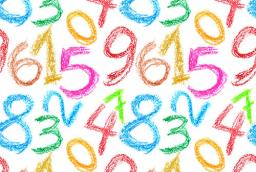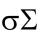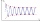# Digit sum

Find the smallest natural number n, the digit sum of which is equal to 37.

n =  19999

### Step-by-step explanation:Did you find an error or inaccuracy? Feel free to write us. Thank you!## Related math problems and questions:

• Three numbersCreate from digits 1-9 three-digit numbers with their sum the smallest. What value is the sum of these numbers? (Use each digit only once)
• The sumThe sum of five consecutive odd numbers is 75. Find out the sum of the second and fourth of them.
• Digit sumDetermine for how many integers greater than 900 and less than 1,001 has digit sum digit of the digit sum number 1.
• Two-digit numberDigit sum of thinking two-digit natural number is 11. When it exchanging a sequence of digits, given a number which is 27 less than the thinking number. Find out which number I think.
• Unknown numberFind the unknown number equal to a quarter of a fifth of a number, which is by 152 more than an unknown number.
• NumberWhat number should be placed instead of the asterisk in number 702*8 to get a number divisible by 6?
• How manyHow many numbers are less than 222 with a digit sum is 8?
• Four-digit numberFind also a four-digit number, which quadrupled written backwards is the same number.
• DivisibilityDetermine all divisors of number 84.
• Common divisorsFind all common divisors of numbers 30 and 45.
• Number with onesThe first digit of the number is 1, if we move this digit to the end we get a 3 times higher number, which is the number?
• Divisible by nineHow many three-digit natural numbers in total are divisible without a remainder by the number 9?
• Sum of four numbersThe sum of four consecutive natural numbers is 114. Find them.
• Closest natural numberFind the closest natural number to 4.456 to 44.56 and 445.6.
• 7 digit numberIf 3c54d10 is divisible by 330, what is the sum of c and d?
• Counting numberWhat is the smallest counting number divisible by 2,5,7,8 and 15?
• Find unknown numberWhat is the number between 50 and 55 that is divisible by 2,3,6,9?